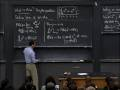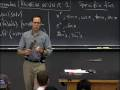Session 18: Derivatives of other Exponential Functions« Previous | Next »

Overview

This session introduces the technique of logarithmic differentiation and uses it to find the derivative of a^x. Substituting different values for a yields formulas for the derivatives of several important functions. Further applications of logarithmic differentiation include verifying the formula for the derivative of x^r, where r is any real number.

Lecture Video and Notes

Video Excerpts

Flash and JavaScript are required for this feature.» Clip 1: Other Bases (00:01:00)

From Lecture 6 of 18.01 Single Variable Calculus, Fall 2006

Flash and JavaScript are required for this feature.» Clip 2: Derivative of ax, Logarithmic (00:05:00)

From Lecture 6 of 18.01 Single Variable Calculus, Fall 2006

Flash and JavaScript are required for this feature.» Clip 3: Derivative of xx (00:03:00)

From Lecture 6 of 18.01 Single Variable Calculus, Fall 2006

Recitation Video

Rules of Logs

Flash and JavaScript are required for this feature.

Lecture Video and Notes

Video Excerpts

Flash and JavaScript are required for this feature.» Clip 1: The Power Rule (00:04:00)

From Lecture 7 of 18.01 Single Variable Calculus, Fall 2006

« Previous | Next »